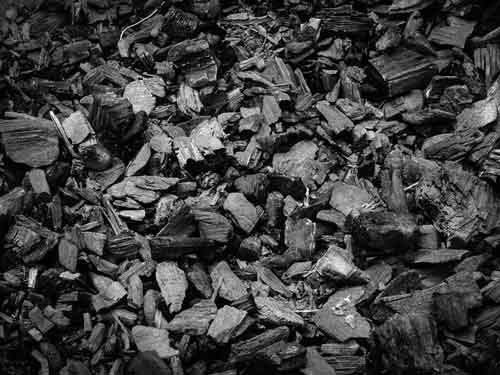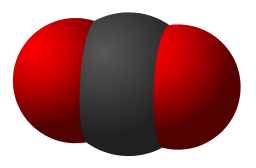Q1-4: Charcoal is made of carbon. When charcoal burns, it combines with oxygen to make carbon dioxide.1. Which row in this table correctly describes carbon and carbon dioxide?

 carbon carbon dioxide A element element B element compound C compound element D compound compound

2. Which of the following is the correct word equation for this reaction?

• A. carbon + oxygen carbon dioxide
• B. carbon dioxide carbon + oxygen
• C. carbon + carbon dioxide oxygen
• D. carbon dioxide + oxygen carbon

3. Carbon dioxide contains one carbon atom and two oxygen atoms as shown in the image.

Which of these is the correct formula for carbon dioxide?

• A. CO2
• B. CO2
• C. C2O
• D. CO2Jacek FH | CC-BY-SA 3.0

4. What is the name given to this type of reaction?

• A. Neutralisation reaction.
• B. Displacement reacton.
• C. Combustion.
• D. Thermal decomposition.

5-7: These questions are about table salt.

Table salt is made of two elements combined: Sodium and chlorine.Salt flats | Bolivia

5. What is the correct chemical name for table salt?

• A. Sodium chlorine
• B. Sodium chloride
• C. Sodium chlorate
• D. Sodium chloroform.

6. Salts can be made when an acid and alkali react as shown here:

 hydrochloric acid + sodium hydroxide → a salt + X (ACID) (ALKALI)

What is the name of substance X?

• A. water
• B. carbon dioxide
• C. sodium chlorine
• D. hydrogen gas

7. What is the name given to the reaction shown in question 6?

• A. Neutralisation reaction.
• B. Displacement reacton.
• C. Combustion.
• D. Thermal decomposition.

8-9. This iron chain has rusted in the open air.8.Which of these sentences is true about the process of an iron chain rusting?

• A. The chain gets lighter as the iron reacts with oxygen in the air.
• B. The chain gets heavier as the iron reacts with oxygen in the air.
• C. The chain gets lighter as the iron reacts with nitrogen in the air.
• D. The chain gets heavier as the iron reacts with nitrogen in the air.

9. What is the name given to this kind of reaction?

• A. Neutralisation reaction.
• B. Displacement reacton.
• C. Oxidation.
• D. Combustion.